# DATA STRUCTURES unit 1.pptx

15 de Nov de 2022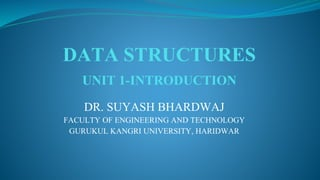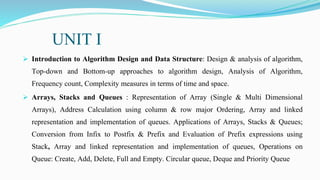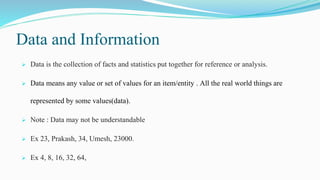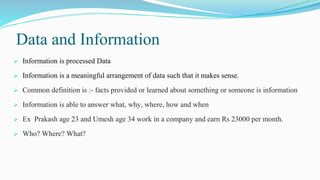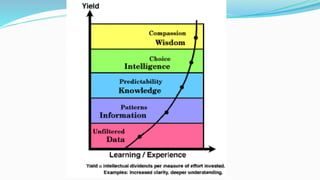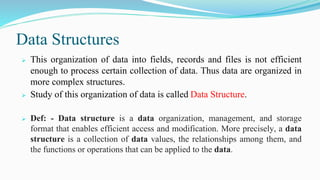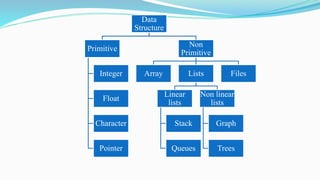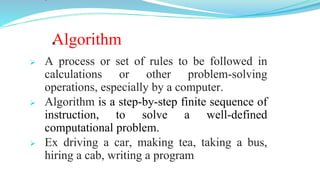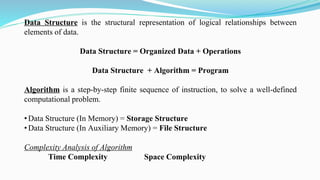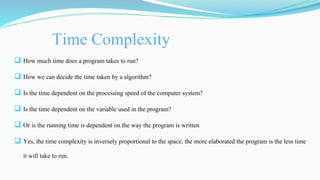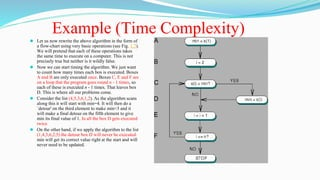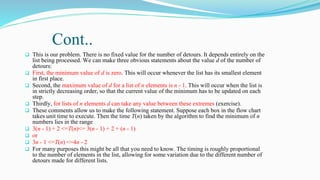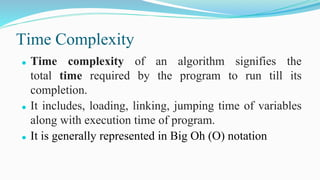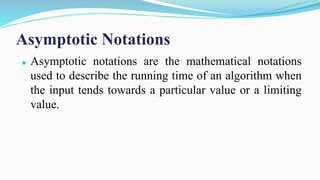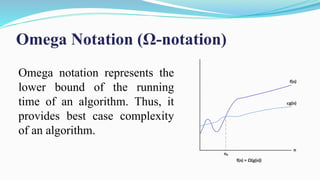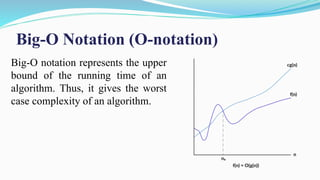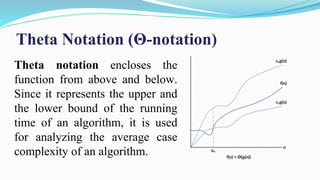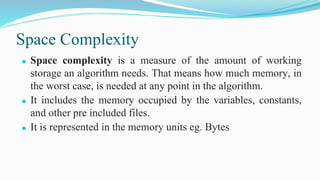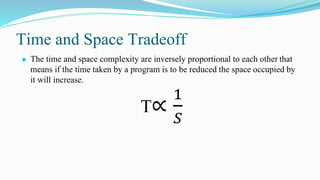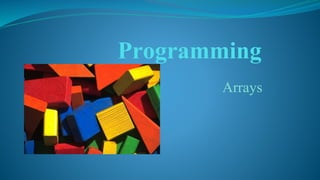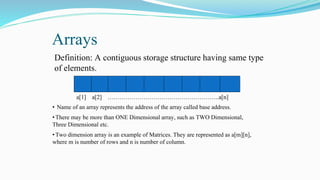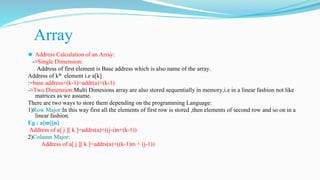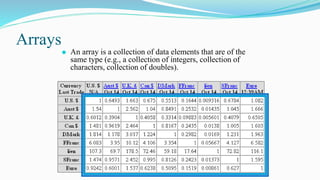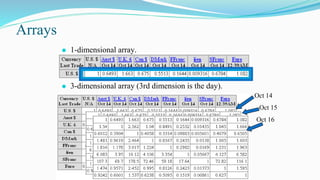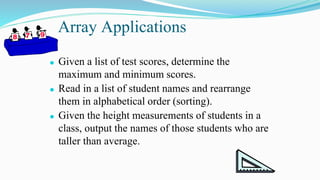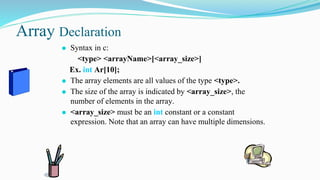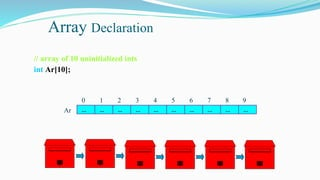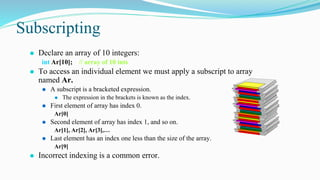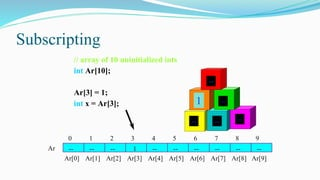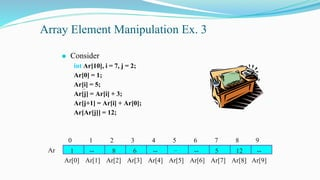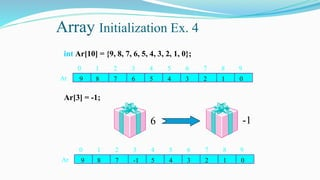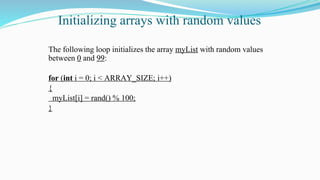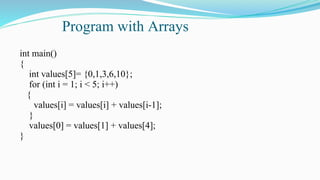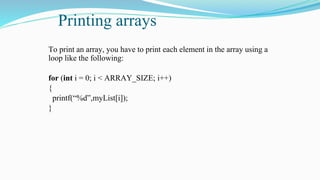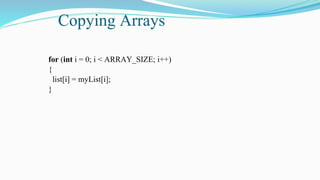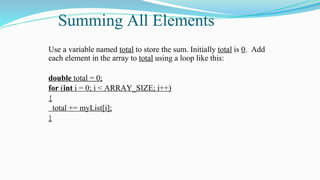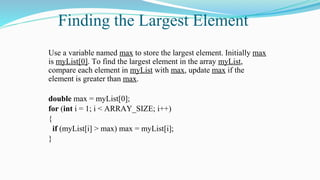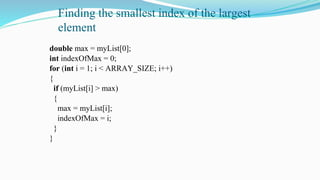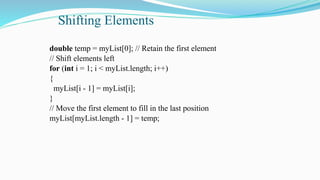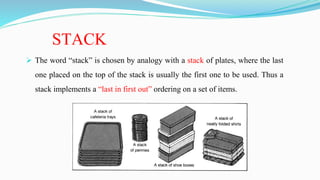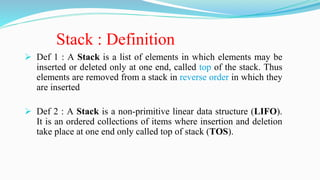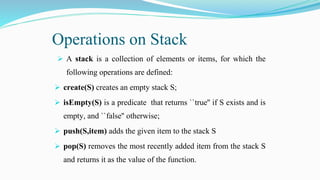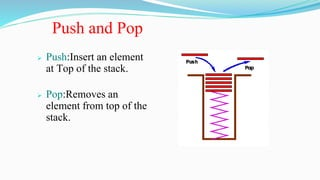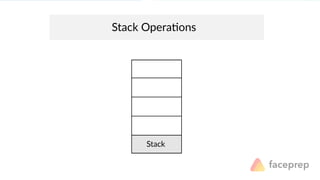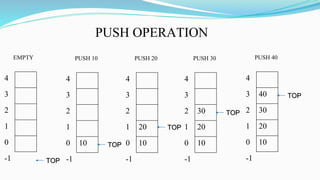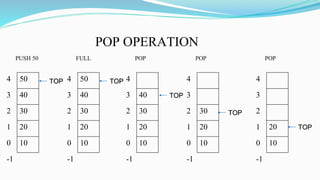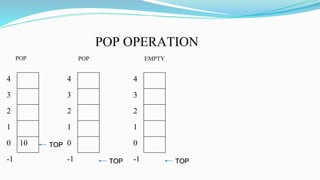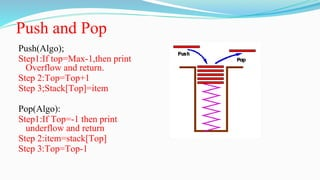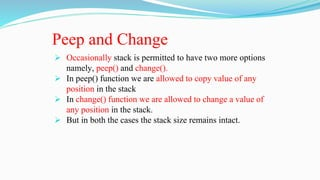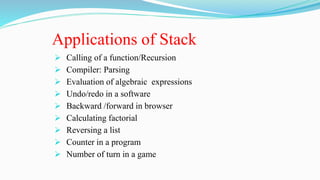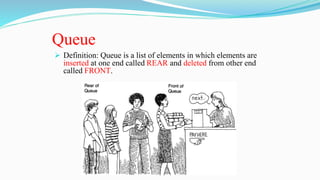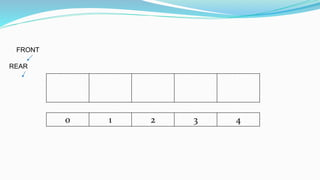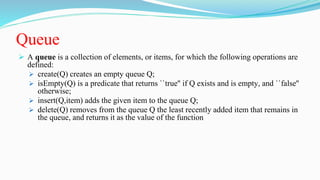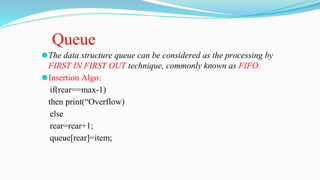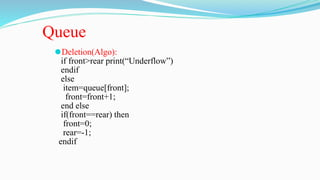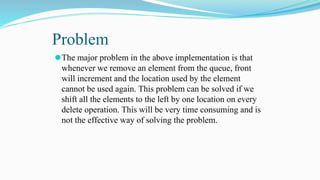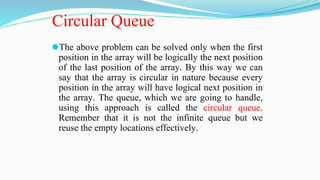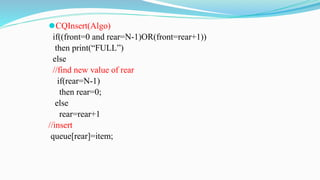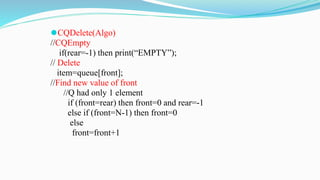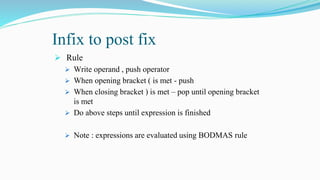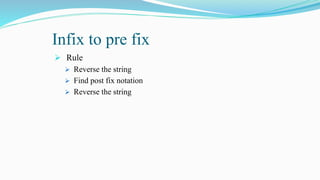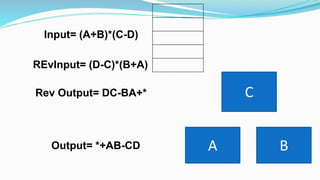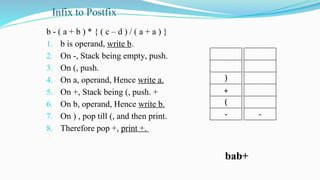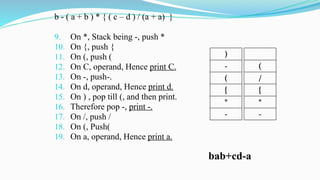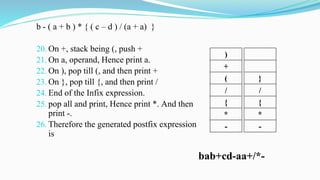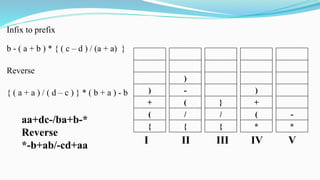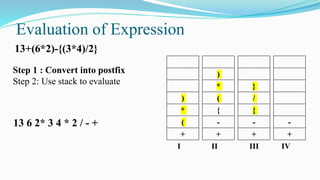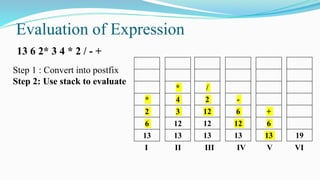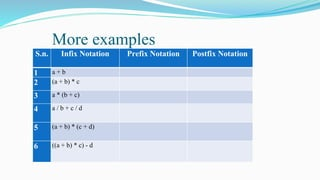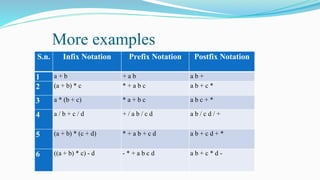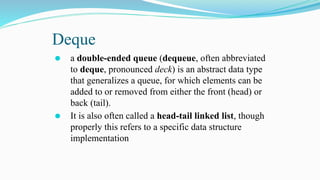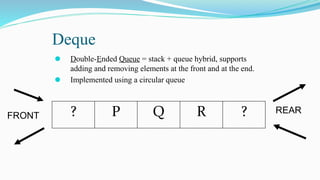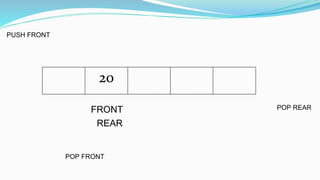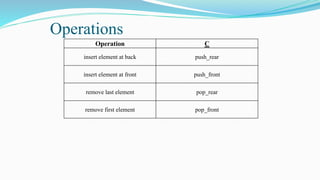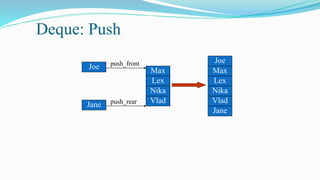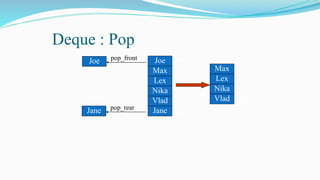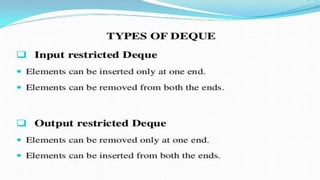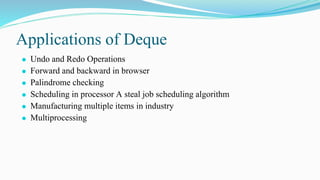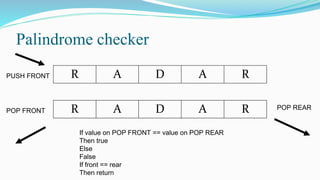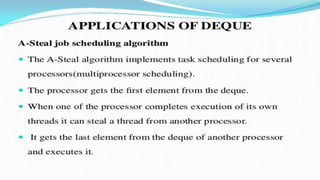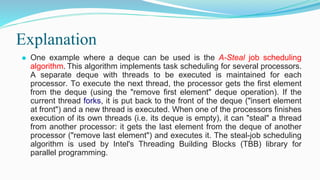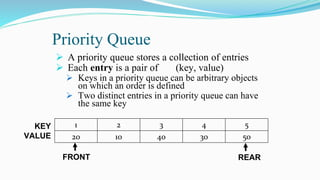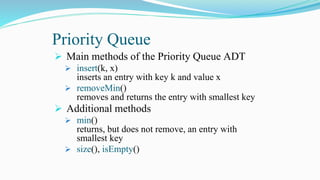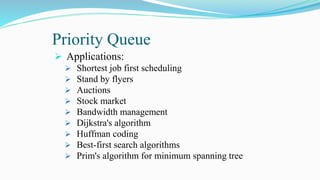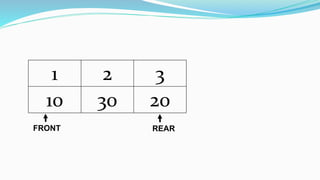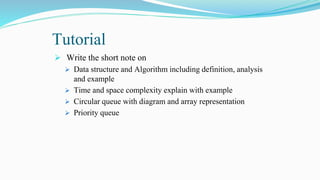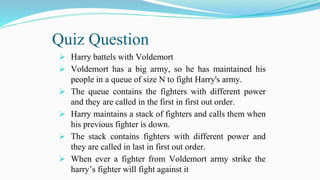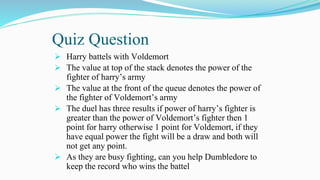1 de 91

### DATA STRUCTURES unit 1.pptx

• 1. DATA STRUCTURES UNIT 1-INTRODUCTION DR. SUYASH BHARDWAJ FACULTY OF ENGINEERING AND TECHNOLOGY GURUKUL KANGRI UNIVERSITY, HARIDWAR
• 2. UNIT I  Introduction to Algorithm Design and Data Structure: Design & analysis of algorithm, Top-down and Bottom-up approaches to algorithm design, Analysis of Algorithm, Frequency count, Complexity measures in terms of time and space.  Arrays, Stacks and Queues : Representation of Array (Single & Multi Dimensional Arrays), Address Calculation using column & row major Ordering, Array and linked representation and implementation of queues. Applications of Arrays, Stacks & Queues; Conversion from Infix to Postfix & Prefix and Evaluation of Prefix expressions using Stack, Array and linked representation and implementation of queues, Operations on Queue: Create, Add, Delete, Full and Empty. Circular queue, Deque and Priority Queue
• 3. Data and Information  Data is the collection of facts and statistics put together for reference or analysis.  Data means any value or set of values for an item/entity . All the real world things are represented by some values(data).  Note : Data may not be understandable  Ex 23, Prakash, 34, Umesh, 23000.  Ex 4, 8, 16, 32, 64,
• 4. Data and Information  Information is processed Data  Information is a meaningful arrangement of data such that it makes sense.  Common definition is :- facts provided or learned about something or someone is information  Information is able to answer what, why, where, how and when  Ex Prakash age 23 and Umesh age 34 work in a company and earn Rs 23000 per month.  Who? Where? What?
• 6. Data Structures  This organization of data into fields, records and files is not efficient enough to process certain collection of data. Thus data are organized in more complex structures.  Study of this organization of data is called Data Structure.  Def: - Data structure is a data organization, management, and storage format that enables efficient access and modification. More precisely, a data structure is a collection of data values, the relationships among them, and the functions or operations that can be applied to the data.
• 8. Algorithm  A process or set of rules to be followed in calculations or other problem-solving operations, especially by a computer.  Algorithm is a step-by-step finite sequence of instruction, to solve a well-defined computational problem.  Ex driving a car, making tea, taking a bus, hiring a cab, writing a program
• 9. Data Structure is the structural representation of logical relationships between elements of data. Data Structure = Organized Data + Operations Data Structure + Algorithm = Program Algorithm is a step-by-step finite sequence of instruction, to solve a well-defined computational problem. • Data Structure (In Memory) = Storage Structure • Data Structure (In Auxiliary Memory) = File Structure Complexity Analysis of Algorithm Time Complexity Space Complexity
• 10. Time and Space Complexity
• 11. Time Complexity  How much time does a program takes to run?  How we can decide the time taken by a algorithm?  Is the time dependent on the processing speed of the computer system?  Is the time dependent on the variable used in the program?  Or is the running time is dependent on the way the program is written  Yes, the time complexity is inversely proportional to the space, the more elaborated the program is the less time it will take to run.
• 12. Example (Time Complexity) ⚫ Let us now rewrite the above algorithm in the form of a flow-chart using very basic operations (see Fig. 1.7). We will pretend that each of these operations takes the same time to execute on a computer. This is not precisely true but neither is it wildly false. ⚫ Now we can start timing the algorithm. We just want to count how many times each box is executed. Boxes A and B are only executed once. Boxes C, E and F are on a loop that the program goes round n - 1 times, so each of these is executed n - 1 times. That leaves box D. This is where all our problems come. ⚫ Consider the list (4,5,3,6,1,2). As the algorithm scans along this it will start with min=4. It will then do a `detour' on the third element to make min=3 and it will make a final detour on the fifth element to give min its final value of 1. In all the box D gets executed twice. ⚫ On the other hand, if we apply the algorithm to the list (1,4,3,6,2,5) the detour box D will never be executed. min will get its correct value right at the start and will never need to be updated.
• 13. Cont..  This is our problem. There is no fixed value for the number of detours. It depends entirely on the list being processed. We can make three obvious statements about the value d of the number of detours:  First, the minimum value of d is zero. This will occur whenever the list has its smallest element in first place.  Second, the maximum value of d for a list of n elements is n - 1. This will occur when the list is in strictly decreasing order, so that the current value of the minimum has to be updated on each step.  Thirdly, for lists of n elements d can take any value between these extremes (exercise).  These comments allow us to make the following statement. Suppose each box in the flow chart takes unit time to execute. Then the time T(n) taken by the algorithm to find the minimum of n numbers lies in the range  3(n - 1) + 2 <=T(n)<= 3(n - 1) + 2 + (n - 1)  or  3n - 1 <=T(n) <=4n - 2  For many purposes this might be all that you need to know. The timing is roughly proportional to the number of elements in the list, allowing for some variation due to the different number of detours made for different lists.
• 14. Time Complexity ⚫ Time complexity of an algorithm signifies the total time required by the program to run till its completion. ⚫ It includes, loading, linking, jumping time of variables along with execution time of program. ⚫ It is generally represented in Big Oh (O) notation
• 15. Asymptotic Notations ⚫ Asymptotic notations are the mathematical notations used to describe the running time of an algorithm when the input tends towards a particular value or a limiting value.
• 16. Omega Notation (Ω-notation) Omega notation represents the lower bound of the running time of an algorithm. Thus, it provides best case complexity of an algorithm.
• 17. Big-O Notation (O-notation) Big-O notation represents the upper bound of the running time of an algorithm. Thus, it gives the worst case complexity of an algorithm.
• 18. Theta notation encloses the function from above and below. Since it represents the upper and the lower bound of the running time of an algorithm, it is used for analyzing the average case complexity of an algorithm. Theta Notation (Θ-notation)
• 19. Space Complexity ⚫ Space complexity is a measure of the amount of working storage an algorithm needs. That means how much memory, in the worst case, is needed at any point in the algorithm. ⚫ It includes the memory occupied by the variables, constants, and other pre included files. ⚫ It is represented in the memory units eg. Bytes
• 20. Time and Space Tradeoff ⚫ The time and space complexity are inversely proportional to each other that means if the time taken by a program is to be reduced the space occupied by it will increase. T∝ 1 𝑆
• 22. Arrays Definition: A contiguous storage structure having same type of elements. a a ………………………………………………..a[n] • Name of an array represents the address of the array called base address. •There may be more than ONE Dimensional array, such as TWO Dimensional, Three Dimensional etc. •Two dimension array is an example of Matrices. They are represented as a[m][n], where m is number of rows and n is number of column.
• 23. Array ⚫ Address Calculation of an Array: ->Single Dimension: Address of first element is Base address which is also name of the array. Address of kth element i.e a[k] :=base address+(k-1)=addr(a)+(k-1) ->Two Dimension:Multi Dimesions array are also stored sequentially in memory,i.e in a linear fashion not like matrices as we assume. There are two ways to store them depending on the programming Language: 1)Row Major:In this way first all the elements of first row is stored ,then elements of second row and so on in a linear fashion. Eg : a[m][n] Address of a[ j ][ k ]=addrs(a)+((j-i)n+(k-1)) 2)Column Major: Address of a[ j ][ k ]=addrs(a)+((k-1)m + (j-1))
• 24. Arrays ⚫ An array is a collection of data elements that are of the same type (e.g., a collection of integers, collection of characters, collection of doubles).
• 25. Arrays ⚫ 1-dimensional array. ⚫ 3-dimensional array (3rd dimension is the day). Oct 15 Oct 16 Oct 14
• 26. Array Applications ⚫ Given a list of test scores, determine the maximum and minimum scores. ⚫ Read in a list of student names and rearrange them in alphabetical order (sorting). ⚫ Given the height measurements of students in a class, output the names of those students who are taller than average.
• 27. Array Declaration ⚫ Syntax in c: <type> <arrayName>[<array_size>] Ex. int Ar; ⚫ The array elements are all values of the type <type>. ⚫ The size of the array is indicated by <array_size>, the number of elements in the array. ⚫ <array_size> must be an int constant or a constant expression. Note that an array can have multiple dimensions.
• 28. Array Declaration // array of 10 uninitialized ints int Ar; -- -- -- -- Ar -- -- -- -- -- -- 4 5 6 3 0 2 8 9 7 1 0 1 2 3 4 5
• 29. Subscripting ⚫ Declare an array of 10 integers: int Ar; // array of 10 ints ⚫ To access an individual element we must apply a subscript to array named Ar. ⚫ A subscript is a bracketed expression. ⚫ The expression in the brackets is known as the index. ⚫ First element of array has index 0. Ar ⚫ Second element of array has index 1, and so on. Ar, Ar, Ar,… ⚫ Last element has an index one less than the size of the array. Ar ⚫ Incorrect indexing is a common error.
• 30. Subscripting // array of 10 uninitialized ints int Ar; Ar = 1; int x = Ar; -- -- 1 -- Ar -- -- -- -- -- -- 4 5 6 3 0 2 8 9 7 1 Ar Ar Ar Ar Ar Ar Ar Ar Ar Ar 1 -- -- -- -- --
• 31. Array Element Manipulation Ex. 3 ⚫ Consider int Ar, i = 7, j = 2; Ar = 1; Ar[i] = 5; Ar[j] = Ar[i] + 3; Ar[j+1] = Ar[i] + Ar; Ar[Ar[j]] = 12; -- 8 6 1 Ar -- 5 12 -- 4 5 6 3 0 2 8 9 7 1 Ar Ar Ar Ar Ar Ar Ar Ar Ar Ar -- -- --
• 32. Array Initialization Ex. 4 int Ar = {9, 8, 7, 6, 5, 4, 3, 2, 1, 0}; Ar = -1; 8 7 6 9 Ar 4 3 2 5 1 0 4 5 6 3 0 2 8 9 7 1 8 7 -1 9 Ar 4 3 2 5 1 0 4 5 6 3 0 2 8 9 7 1 6 -1
• 33. Initializing arrays with random values The following loop initializes the array myList with random values between 0 and 99: for (int i = 0; i < ARRAY_SIZE; i++) { myList[i] = rand() % 100; }
• 34. Program with Arrays int main() { int values= {0,1,3,6,10}; for (int i = 1; i < 5; i++) { values[i] = values[i] + values[i-1]; } values = values + values; }
• 35. Printing arrays To print an array, you have to print each element in the array using a loop like the following: for (int i = 0; i < ARRAY_SIZE; i++) { printf(“%d”,myList[i]); }
• 36. Copying Arrays for (int i = 0; i < ARRAY_SIZE; i++) { list[i] = myList[i]; }
• 37. Summing All Elements Use a variable named total to store the sum. Initially total is 0. Add each element in the array to total using a loop like this: double total = 0; for (int i = 0; i < ARRAY_SIZE; i++) { total += myList[i]; }
• 38. Finding the Largest Element Use a variable named max to store the largest element. Initially max is myList. To find the largest element in the array myList, compare each element in myList with max, update max if the element is greater than max. double max = myList; for (int i = 1; i < ARRAY_SIZE; i++) { if (myList[i] > max) max = myList[i]; }
• 39. Finding the smallest index of the largest element double max = myList; int indexOfMax = 0; for (int i = 1; i < ARRAY_SIZE; i++) { if (myList[i] > max) { max = myList[i]; indexOfMax = i; } }
• 40. Shifting Elements double temp = myList; // Retain the first element // Shift elements left for (int i = 1; i < myList.length; i++) { myList[i - 1] = myList[i]; } // Move the first element to fill in the last position myList[myList.length - 1] = temp;
• 41. STACK
• 42. STACK  The word “stack” is chosen by analogy with a stack of plates, where the last one placed on the top of the stack is usually the first one to be used. Thus a stack implements a “last in first out” ordering on a set of items.
• 43. Stack : Definition  Def 1 : A Stack is a list of elements in which elements may be inserted or deleted only at one end, called top of the stack. Thus elements are removed from a stack in reverse order in which they are inserted  Def 2 : A Stack is a non-primitive linear data structure (LIFO). It is an ordered collections of items where insertion and deletion take place at one end only called top of stack (TOS).
• 44. Operations on Stack  A stack is a collection of elements or items, for which the following operations are defined:  create(S) creates an empty stack S;  isEmpty(S) is a predicate that returns ``true'' if S exists and is empty, and ``false'' otherwise;  push(S,item) adds the given item to the stack S  pop(S) removes the most recently added item from the stack S and returns it as the value of the function.
• 45. Push and Pop  Push:Insert an element at Top of the stack.  Pop:Removes an element from top of the stack.
• 47. 4 3 2 1 0 -1 TOP EMPTY 10 4 3 2 1 0 -1 TOP PUSH 10 20 10 4 3 2 1 0 -1 TOP PUSH 20 30 20 10 4 3 2 1 0 -1 TOP PUSH 30 PUSH OPERATION 40 30 20 10 4 3 2 1 0 -1 TOP PUSH 40
• 50. Push and Pop Push(Algo); Step1:If top=Max-1,then print Overflow and return. Step 2:Top=Top+1 Step 3;Stack[Top]=item Pop(Algo): Step1:If Top=-1 then print underflow and return Step 2:item=stack[Top] Step 3:Top=Top-1
• 51. Peep and Change  Occasionally stack is permitted to have two more options namely, peep() and change().  In peep() function we are allowed to copy value of any position in the stack  In change() function we are allowed to change a value of any position in the stack.  But in both the cases the stack size remains intact.
• 52. Applications of Stack  Calling of a function/Recursion  Compiler: Parsing  Evaluation of algebraic expressions  Undo/redo in a software  Backward /forward in browser  Calculating factorial  Reversing a list  Counter in a program  Number of turn in a game
• 53. Queue  Definition: Queue is a list of elements in which elements are inserted at one end called REAR and deleted from other end called FRONT.
• 55. Queue  A queue is a collection of elements, or items, for which the following operations are defined:  create(Q) creates an empty queue Q;  isEmpty(Q) is a predicate that returns ``true'' if Q exists and is empty, and ``false'' otherwise;  insert(Q,item) adds the given item to the queue Q;  delete(Q) removes from the queue Q the least recently added item that remains in the queue, and returns it as the value of the function
• 56. ⚫The data structure queue can be considered as the processing by FIRST IN FIRST OUT technique, commonly known as FIFO. ⚫Insertion Algo: if(rear==max-1) then print(“Overflow) else rear=rear+1; queue[rear]=item; Queue
• 58. Problem ⚫The major problem in the above implementation is that whenever we remove an element from the queue, front will increment and the location used by the element cannot be used again. This problem can be solved if we shift all the elements to the left by one location on every delete operation. This will be very time consuming and is not the effective way of solving the problem.
• 59. Circular Queue ⚫The above problem can be solved only when the first position in the array will be logically the next position of the last position of the array. By this way we can say that the array is circular in nature because every position in the array will have logical next position in the array. The queue, which we are going to handle, using this approach is called the circular queue. Remember that it is not the infinite queue but we reuse the empty locations effectively.
• 60. ⚫CQInsert(Algo) if((front=0 and rear=N-1)OR(front=rear+1)) then print(“FULL”) else //find new value of rear if(rear=N-1) then rear=0; else rear=rear+1 //insert queue[rear]=item;
• 61. ⚫CQDelete(Algo) //CQEmpty if(rear=-1) then print(“EMPTY”); // Delete item=queue[front]; //Find new value of front //Q had only 1 element if (front=rear) then front=0 and rear=-1 else if (front=N-1) then front=0 else front=front+1
• 63. Infix to post fix  Rule  Write operand , push operator  When opening bracket ( is met - push  When closing bracket ) is met – pop until opening bracket is met  Do above steps until expression is finished  Note : expressions are evaluated using BODMAS rule
• 64. Infix to pre fix  Rule  Reverse the string  Find post fix notation  Reverse the string
• 65. Input= (A+B)*(C-D) Rev Output= DC-BA+* REvInput= (D-C)*(B+A) Output= *+AB-CD A B C
• 66. Infix to Postfix b - ( a + b ) * { ( c – d ) / ( a + a ) } 1. b is operand, write b. 2. On -, Stack being empty, push. 3. On (, push. 4. On a, operand, Hence write a. 5. On +, Stack being (, push. + 6. On b, operand, Hence write b. 7. On ) , pop till (, and then print. 8. Therefore pop +, print +. ) + ( - bab+ -
• 67. b - ( a + b ) * { ( c – d ) / (a + a) } 9. On *, Stack being -, push * 10. On {, push { 11. On (, push ( 12. On C, operand, Hence print C. 13. On -, push-. 14. On d, operand, Hence print d. 15. On ) , pop till (, and then print. 16. Therefore pop -, print -. 17. On /, push / 18. On (, Push( 19. On a, operand, Hence print a. ) - ( { * - bab+cd-a ( / { * -
• 68. b - ( a + b ) * { ( c – d ) / (a + a) } 20. On +, stack being (, push + 21. On a, operand, Hence print a. 22. On ), pop till (, and then print + 23. On }, pop till {, and then print / 24. End of the Infix expression. 25. pop all and print, Hence print *. And then print -. 26. Therefore the generated postfix expression is ) + ( / { * - bab+cd-aa+/*- } / { * -
• 69. Infix to prefix b - ( a + b ) * { ( c – d ) / (a + a) } Reverse { ( a + a ) / ( d – c ) } * ( b + a ) - b ) + ( { aa+dc-/ba+b-* Reverse *-b+ab/-cd+aa } / { ) - ( / { ) + ( * I II III IV V - *
• 70. Evaluation of Expression ) * ( + } / { - + ) * ( { - + - + I II III IV 13+(6*2)-{(3*4)/2} Step 1 : Convert into postfix Step 2: Use stack to evaluate 13 6 2* 3 4 * 2 / - +
• 71. Evaluation of Expression * 2 6 13 / 2 12 12 13 * 4 3 12 13 - 6 12 13 I II III IV V VI Step 1 : Convert into postfix Step 2: Use stack to evaluate 13 6 2* 3 4 * 2 / - + + 6 13 19
• 72. More examples S.n. Infix Notation Prefix Notation Postfix Notation 1 a + b 2 (a + b) * c 3 a * (b + c) 4 a / b + c / d 5 (a + b) * (c + d) 6 ((a + b) * c) - d
• 73. More examples S.n. Infix Notation Prefix Notation Postfix Notation 1 a + b + a b a b + 2 (a + b) * c * + a b c a b + c * 3 a * (b + c) * a + b c a b c + * 4 a / b + c / d + / a b / c d a b / c d / + 5 (a + b) * (c + d) * + a b + c d a b + c d + * 6 ((a + b) * c) - d - * + a b c d a b + c * d -
• 74. Deque ⚫ a double-ended queue (dequeue, often abbreviated to deque, pronounced deck) is an abstract data type that generalizes a queue, for which elements can be added to or removed from either the front (head) or back (tail). ⚫ It is also often called a head-tail linked list, though properly this refers to a specific data structure implementation
• 75. Deque ⚫ Double-Ended Queue = stack + queue hybrid, supports adding and removing elements at the front and at the end. ⚫ Implemented using a circular queue ? P Q R ? FRONT REAR
• 76. 20 PUSH FRONT POP FRONT POP REAR FRONT REAR
• 77. Operations Operation C insert element at back push_rear insert element at front push_front remove last element pop_rear remove first element pop_front
• 79. Deque : Pop Max Lex Nika Vlad pop_front Joe top end pop_rear Jane Max Lex Nika Vlad top end Joe Jane
• 81. Applications of Deque ⚫ Undo and Redo Operations ⚫ Forward and backward in browser ⚫ Palindrome checking ⚫ Scheduling in processor A steal job scheduling algorithm ⚫ Manufacturing multiple items in industry ⚫ Multiprocessing
• 82. Palindrome checker R A D A R PUSH FRONT R A D A R POP FRONT POP REAR If value on POP FRONT == value on POP REAR Then true Else False If front == rear Then return
• 84. Explanation ⚫ One example where a deque can be used is the A-Steal job scheduling algorithm. This algorithm implements task scheduling for several processors. A separate deque with threads to be executed is maintained for each processor. To execute the next thread, the processor gets the first element from the deque (using the "remove first element" deque operation). If the current thread forks, it is put back to the front of the deque ("insert element at front") and a new thread is executed. When one of the processors finishes execution of its own threads (i.e. its deque is empty), it can "steal" a thread from another processor: it gets the last element from the deque of another processor ("remove last element") and executes it. The steal-job scheduling algorithm is used by Intel's Threading Building Blocks (TBB) library for parallel programming.
• 85. Priority Queue  A priority queue stores a collection of entries  Each entry is a pair of (key, value)  Keys in a priority queue can be arbitrary objects on which an order is defined  Two distinct entries in a priority queue can have the same key 1 2 3 4 5 20 10 40 30 50 KEY VALUE FRONT REAR
• 86. Priority Queue  Main methods of the Priority Queue ADT  insert(k, x) inserts an entry with key k and value x  removeMin() removes and returns the entry with smallest key  Additional methods  min() returns, but does not remove, an entry with smallest key  size(), isEmpty()
• 87. Priority Queue  Applications:  Shortest job first scheduling  Stand by flyers  Auctions  Stock market  Bandwidth management  Dijkstra's algorithm  Huffman coding  Best-first search algorithms  Prim's algorithm for minimum spanning tree
• 88. 1 2 3 10 30 20 FRONT REAR
• 89. Tutorial  Write the short note on  Data structure and Algorithm including definition, analysis and example  Time and space complexity explain with example  Circular queue with diagram and array representation  Priority queue
• 90. Quiz Question  Harry battels with Voldemort  Voldemort has a big army, so he has maintained his people in a queue of size N to fight Harry's army.  The queue contains the fighters with different power and they are called in the first in first out order.  Harry maintains a stack of fighters and calls them when his previous fighter is down.  The stack contains fighters with different power and they are called in last in first out order.  When ever a fighter from Voldemort army strike the harry’s fighter will fight against it
• 91. Quiz Question  Harry battels with Voldemort  The value at top of the stack denotes the power of the fighter of harry’s army  The value at the front of the queue denotes the power of the fighter of Voldemort’s army  The duel has three results if power of harry’s fighter is greater than the power of Voldemort’s fighter then 1 point for harry otherwise 1 point for Voldemort, if they have equal power the fight will be a draw and both will not get any point.  As they are busy fighting, can you help Dumbledore to keep the record who wins the battel